# NCERT Solutions - Determinants, Exercise 4.3 JEE Notes | EduRev

## JEE : NCERT Solutions - Determinants, Exercise 4.3 JEE Notes | EduRev

The document NCERT Solutions - Determinants, Exercise 4.3 JEE Notes | EduRev is a part of the JEE Course Mathematics (Maths) Class 12.
All you need of JEE at this link: JEE

Determinants

Exercise 4.3

Question 1:
Find area of the triangle with vertices at the point given in each of the following:
(i) (1, 0), (6, 0), (4, 3)
(ii) (2, 7), (1, 1), (10, 8)
(iii) (−2, −3), (3, 2), (−1, −8)

(i) The area of the triangle with vertices (1, 0), (6, 0), (4, 3) is given by the relation,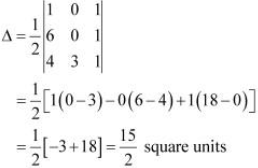(ii) The area of the triangle with vertices (2, 7), (1, 1), (10, 8) is given by the relation,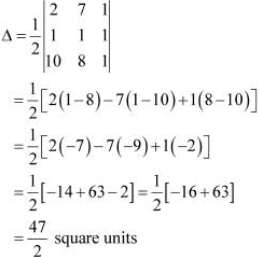(iii)The area of the triangle with vertices (−2, −3), (3, 2), (−1, −8) is given by the relation,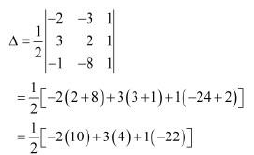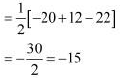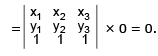Hence, the area of the triangle is |-15| = 15 sq units

Question 2: Show that points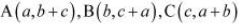are collinear

Area of ΔABC is given by the relation,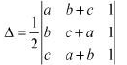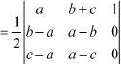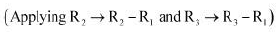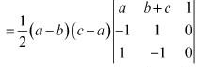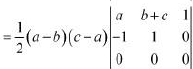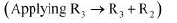= 0

Thus, the area of the triangle formed by points A, B, and C is zero. Hence, the points A, B, and C are collinear.

Question 3: Find values of k if area of triangle is 4 square units and vertices are
(i) (k, 0), (4, 0), (0, 2)

(ii) (−2, 0), (0, 4), (0, k)

We know that the area of a triangle whose vertices are (x1, y1), (x2, y2), and (x3, y3) is the absolute value of the determinant (Δ), where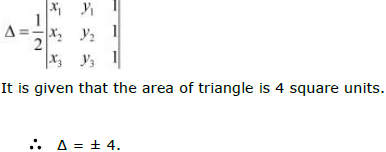(i) The area of the triangle with vertices (k, 0), (4, 0), (0, 2) is given by the relation,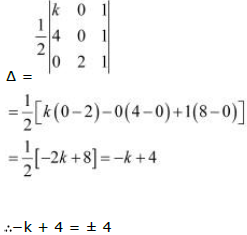When −k + 4 = − 4, k = 8.
When −k + 4 = 4, k = 0.
Hence, k = 0, 8.

(ii) The area of the triangle with vertices (−2, 0), (0, 4), (0, k) is given by the relation,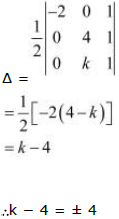When k − 4 = − 4, k = 0.
When k − 4 = 4, k = 8.
Hence, k = 0, 8.

Question 4:
(i) Find equation of line joining (1, 2) and (3, 6) using determinants
(ii) Find equation of line joining (3, 1) and (9, 3) using determinants

(i) Let P (x, y) be any point on the line joining points A (1, 2) and B (3, 6). Then, the points A, B, and P are collinear. Therefore, the area of triangle ABP will be zero.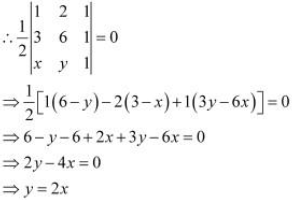Hence, the equation of the line joining the given points is y = 2x.
(ii) Let P (x, y) be any point on the line joining points A (3, 1) and B (9, 3). Then, the points A, B, and P are collinear. Therefore, the area of triangle ABP will be zero.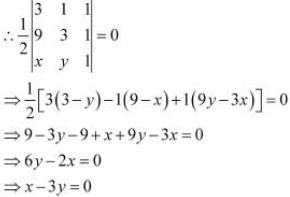Hence, the equation of the line joining the given points is x − 3y = 0.

Question 5: If area of triangle is 35 square units with vertices (2, −6), (5, 4), and (k, 4). Then k is
A. 12

B. −2

C. −12, −2

D. 12, −2

The area of the triangle with vertices (2, −6), (5, 4), and (k, 4) is given by the relation,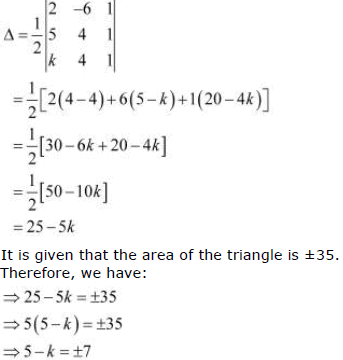When 5 − k = −7, k = 5 + 7 = 12.
When 5 − k = 7, k = 5 − 7 = −2.
Hence, k = 12, −2.

Offer running on EduRev: Apply code STAYHOME200 to get INR 200 off on our premium plan EduRev Infinity!

## Mathematics (Maths) Class 12

209 videos|222 docs|124 tests

,

,

,

,

,

,

,

,

,

,

,

,

,

,

,

,

,

,

,

,

,

,

,

,

;